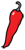The Grand Locus / Life for statistical sciences

## Why p-values are crapI remember my statistics classes as a student. To do a t-test we had to carry out a series of tedious calculations and in the end look up the value in a table. Making those tables cost an enormous amount of sweat from talented statisticians, so you had only three tables, for three significance levels: 5%, 1% and 0.1%. This explains the common way to indicate significance in scientific papers, with one (*), two (**) or three (***) stars. Today, students use computers to do the calcultations so the star notation probably appears as a mysterious folklore and the idea of using a statistical table is properly unthinkable. And this is a good thing because computing those t statistics by hand was a pain. But statistical softwares also paved the way for the invasion of p-values in the scientific literature.

To understand what is wrong with p-values, we will need to go deeper in the theory of statistical testing, so let us review the basic principles. Every statistical test consists of a null hypothesis, a test statistic (a score) and a decision rule — plus the often forgotten alternative hypothesis. A statistical test is an investigation protocol to test if a specific hypothesis is true or false. The outcome of a test is always a yes/no answer.

The decision rule of a test depends on a crucial parameter which is the level or significance. By definition, the level is the risk of false positive (or type I error) that you accept. The p-value of a test is the maximum false positive risk you would take by rejecting the null hypothesis. For instance, if the p-value of a test is 0.034, you have a 3.4% chance of being wrong by rejecting the null hypothesis.

With these notions in mind, it is clear that p-values have transformed statistical testing. How often do we hear or read that the results of the test are "very" significant? What is meant here is usually that the p-value is very low. But does this mean that the null hypothesis is very false?

That is what I came to believe after spending some time with my first datasets. I thought that there are only two ways a p-value can be low: either the sample is large, or the effect size is very strong. For instance if you had showed me the results of two t-tests performed on samples of identical size, and that the first p-value was 0.0001 and the second 0.001, I would have said that the mean/variance ratio of the first population is larger. Actually this is completely wrong.

To build a counter example, we will take a Brownian motion, which we introduced previously. The distribution of the standard Brownian motion is Gaussian at every time point, and centered around 0. Let's emphasize this because this is key to what follows: by construction, the brownian motion has zero mean. The only way the null hypothesis is violated is by the fact that observations are not IID (independent and identically distributed).

The following techical section shows how to perform a t-test on the observations of a Brownian motion with R. We build a series of random variables that all have a standard Gaussian distributions but are not independent — because they consist of cumulative sums of Gaussian variables. In those conditions, the mean is still an unbiased estimator, but its variance is no longer $1/n$ (where $n$ is the sample size). Since the variance $1/n$ is central to the distribution of the $t$ statistic, the results are off, which leads to huge rejection rates. This is what should happen because the null hypoythesis is false, but the effect size is 0.

Here are some lines in R. You can copy-paste the whole thing in your R session.

rBrownian <- function(n) {   # Simulate n observations of a Brownian motion   # equally spaced between 0 and 1. Levy's construction   # that we showed in previous post is more elegant but   # harder to implement. Here we simply compute the   # cumulative sum of Gaussian variables.   cumsum(rnorm(n, sd=sqrt(1/n)))}# This should be significant.t.test(rBrownian(100))# The observation at time t has variance t.# We can stabilize the variance by dividing by 'sqrt(t)', in which# case all the variables are Gaussian (0,1) but not independent.rBrownianID <- function(n) {   cumsum(rnorm(n, sd=sqrt(1/n))) / sqrt(seq(1,n)/n)}    t.test(rBrownianID(100))# Be extra careful here. For example the 'sd' function will give# you a very biased estimate of the standard deviation. This is# because the estimate is unbiased only under the assumption# of independence.sd(rBrownianID(100))# Now show that p-values are skewed.p.vals.H0_true <- rep(NA, 10000)p.vals.Brownian <- rep(NA, 10000)for (i in 1:10000) {   p.vals.H0_true[i] <- t.test(rnorm(100))$p.value p.vals.Brownian[i] <- t.test(rBrownianID(100))$p.value}plot(sort(p.vals.H0_true), type='l', ylab='p-value')lines(sort(p.vals.Brownian), col='red')# The variables are Gaussian (0,1) by construction so the effect size# is null, and yet...median(p.vals.Brownian)

In conclusion, low p-values tell us nothing about the effect size. A low p-value only says that you should reject the null hypothesis. Lack of independence is enough to drive your p-values below the ground. Now, take a moment to think about the last test you performed, and ask yourself whether sampling was independent.

But then what should we use instead? I have to admit that I have no practical alternative to offer. Even more so because the situation is not bad: it is hopeless. It is statistical testing that is wrong, not just p-values: rejecting the null hypothesis $\mu = 0$ (the mean of the population is zero) does not mean that $\mu \neq 0$. It means that either $\mu \neq 0$ or that sampling was not perfectly independent or that it did not have the distribution you assumed. So the best you can do is remember that it is practically impossible to prove anything with statistics. One can say that there is something wrong with the null hypothesis, but it is hard to know what that is.